# Spontaneous mirror symmetry breaking: an entropy production survey of the racemate instability and the emergence of stable scalemic stationary states†

Josep M. Ribó*ab and David Hochberg*c
aDepartment of Organic Chemistry, University of Barcelona, E-08028 Barcelona, Catalonia, Spain. E-mail: jmribo@ub.edu
bInstitute of Cosmos Science (IEEC-UB), University of Barcelona, E-08028 Barcelona, Catalonia, Spain
cDepartment of Molecular Evolution, Centro de Astrobiology (CSIC-INTA), E-28850 Torrejón de Ardoz, Madrid, Spain. E-mail: hochbergd@cab.inta-csic.es

Received 28th April 2020 , Accepted 8th June 2020

First published on 8th June 2020

We study the emergence of both stable and unstable non-equilibrium stationary states (NESS), as well as spontaneous mirror symmetry breaking (SMSB) provoked by the destabilization of the racemic thermodynamic branch, for an enantioselective autocatalytic reaction network in an open flow system, and for a continuous range n of autocatalytic orders. The system possesses a range of double bi-stability and also tri-stability depending on the autocatalytic order. We carry out entropy production and entropy flow calculations, from simulations of ordinary differential equations, stoichiometric network analysis (SNA), and consider a stability analysis of the NESS. The simulations provide a correct description of the relationship between energy state functions, the isothermal dissipated heat, entropy production and entropy flow exchange with the surroundings, and the correct solution of the balance of the entropy currents at the NESS. The validity of the General Evolution Criterion (GEC) is in full agreement with all the dynamic simulations.

## Introduction

Explanations of the biological homochirality (BH) phenomenon, based on scenarios of kinetic control of irreversible reactions subject to initial stochastic enantiomeric excesses,1,2 do not substantiate the resilience of real biochemical networks to racemization. BH would be sustained by the behaviour of enantioselective autocatalytic networks working in open systems:3–6 Enantioselective autocatalysis is the necessary reaction that supports the existence of biases away from the racemic composition, and this shares a significant analogy with the role of autocatalysis as a principal characteristic of life.7–10 Biochemical reaction networks are based on the coexistence of, and cooperative effects between, non-equilibrium steady states (NESS), which can retain their relative stabilities within a range of boundary conditions and time domains.11,12 In this respect, recent speculative scenarios on BH have analogies with proposals for the emergence of autocatalytic sets during the chemical evolution stages of compartmentalization and the synthesis of functionalized polymers supporting specific catalytic functions and self-reproduction.7,8,13 A reasonable speculation for the chemical basis of BH is the emergence of non-equilibrium steady states (NESS) with biases away from the racemic composition (scalemic mixtures). This implies a bifurcation scenario where the thermodynamic branch of the racemic NESS is destabilized, and the bistability of an enantiomeric pair of stable NESS's emerges.

Deracemization is an extreme case of chemical selection, by virtue of the energetic degeneracy between the pair of enantiomers. In the absence of external chiral polarizations, biases away from the racemic composition may be obtained in highly non-linear enantioselective autocatalytic networks, when these operate in open systems, or in closed systems with inhomogeneous distributions of matter and or energy.14 In closed systems showing isotropic energy and mass distribution, the final state must necessarily be the racemate, however, in them, for highly exergonic transformations, temporary scalemic time windows or chiral excursions may be achieved.15 It is worth noting that most of the transformations originating within complex biochemical networks are, in fact, low exergonic transformations,16, therefore, “reversible” reactions should be taken into account also in the description of the irreversibility of open systems. A general explanation of BH should be based on scenarios of open systems, or on systems whose boundary conditions prevent the system from achieving thermodynamic equilibrium. In summary, the study of BH and spontaneous mirror symmetry breaking (SMSB) belongs to the framework of irreversible thermodynamics. Furthermore, the simple racemization/deracemization reaction already implies a change of the configurational entropy leading to affinity (Af) values that do not fulfil the condition of linear-regime thermodynamics, that is, do not satisfy.17,18 This means that a description of SMSB must be Af/RT ≪ 1 made in the framework of the non-linear regime of irreversible thermodynamics.

The thermodynamic framework for the study of such dissipative systems was pioneered and developed, already fifty years ago, specially by the Brussels school.19–21 In this scenario, the entropy production may reach a critical value where the thermodynamic branch (corresponding to the racemic NESS in the case of SMSB)3 is destabilized, and new stable NESS's having biases away from the racemic composition can appear. However, researchers have traditionally shown a preferential interest for the modelling and simulation of spatial dissipative structures, rather than the analysis of the NESS's of reactions in homogeneous and isotropic media. Furthermore, the relative unavailability, up to only a few years ago, of computers and software for solving numerically the differential equation sets of chemical reactions, led to the use of certain older approximations used in chemical kinetics, which overlooked the thermodynamic constraints imposed by the boundary conditions. Such approximations and simplifications run the risk of leading to incomplete, if not simply erroneous, interpretations of system thermodynamics. Regarding SMSB, the previous statements of the Brussels school concerning systems leading to bifurcations of the thermodynamic branch are significant (see ref. 20, Chapter XVI): these are that (a) the entropy production of a catalytic transformation is greater than that of a non-catalytic one, and (b) the necessity, but not the sufficiency, of enantioselective autocatalysis.

In this paper we report how enantioselective autocatalysis leads to SMSB in open systems, and in the non-linear regime of irreversible thermodynamics, depending on the autocatalytic order n. Stoichiometric network analysis (SNA), polynomial root solvers and dynamic simulations of the differential rate equations allow us to carry out a detailed analysis of the entropy production and the entropy flow with regards to the emergence of SMSB, that is, the bifurcation from the racemic thermodynamic branch towards stable scalemic NESS with large enantiomeric excess (ee) values. The results reveal SMSB as paradigm of the principles governing the formation of multiple stationary states in dissipative reaction systems, and they exemplify and underscore the significance of the General Evolution Criterion (GEC).21–23

## Methodology

### Model

Replicators in systems biology are autocatalytic assemblies capable of self-reproduction and the transmission of information.24–26 The reductionist representation of a chemical replicator R is
 A + nR ⇆ (n + 1)R, (1)
where A represents the resources for forming R. The reaction (1) in a chemical network must be represented as a reversible transformation, because the rate constants of the forward and backward reaction steps are necessary for applying the thermodynamic constraints, as determined by the relationship between the equilibrium constants and the reaction rate constants when the direct production of R from A is included.27–29 The actual chemical replicator is constituted by a sequence of bimolecular paths having diverse mechanisms, however, the kinetics expressed in (1) is maintained.

First order autocatalysis [n = 1 in (1)] cannot lead to the preferential selection between replicators that compete for common resources. In systems biology, this corresponds to the growth case known as the “survival of everybody”.30,31 Second order autocatalysis [n = 2 in (1)], may select between replicators. However, kinetic orders higher than the bimolecular are unlikely, because of the low probability of having a simultaneous collision between a number of molecules greater than two. Furthermore, second order autocatalysis, at the low concentrations expected in biological systems, leads to very low chemical yields. It is surely significant that, and to the best of our knowledge, only chemical replicators undergoing first order autocatalysis have been detected.32,33 However, when first order autocatalysis is coupled to other reactions, then effective kinetics equivalent to n > 1 may be achieved31 and, as a consequence, higher selectivity can result between replicators competing for common resources.

Enantioselective autocatalysis implies two enantiomeric chemical replicators. In this respect, in SMSB, the selection between one of the two energetically degenerate enantiomeric replicators, represents an extreme case of chemical selectivity. However, the conditions for this selection are similar to the selection between isomeric or diastereomeric replicators.34,35 The models of reaction networks able to lead to SMSB are constituted by chiral replicators showing first order enantioselective autocatalysis, where the crucial higher non-linearities corresponding to n > 1 are obtained through the coupling with other enantioselective reactions.14 For example, this is achieved in the Frank model by the coupling with a heterochiral reaction4,15,36–38 and in the limited enantio-selective model (LES) by the coupling with the non-enantioselective autocatalysis,39–41 and in the enantioselective hypercycle through additional homochiral cross-catalysis.34,35 We study here enantioselective autocatalysis for different values of n [see below reaction (2)]. The coupling of a transformation with other reactions, such as is the case in biochemical networks, mostly leads to apparent non-integer kinetics.42 A paradigm of this are autocatalytic networks31 and specifically, the previous proposed models on SMSB of first order autocatalyis coupled with other enantioselective reactions. Here, in order to obtain a general description of the effect of the increase of the autocatalytic order n in such SMSB networks, we introduce a reductionist reaction model, to study non-integer values of the autocatalysis (2). The reaction network model also includes the direct non-catalytic synthesis (3) of the chiral replicators D and L. This allows not only for the system to start, even the in the absence of D/L, but more importantly, it allows for testing the resilience of the system to racemization:

 A + nD ⇆ (n + 1)D
 A + nL ⇆ (n + 1)L (k1/k−1). (2)

 A ⇆ D
 A ⇆ L (k0/k−0) (3)
Note, that (2) and (3) have the same equilibrium constant (k0/k−0 = k1/k−1) and that the autocatalytic scenario implies k0k1. The reaction network studied here runs in an open flow reactor allowing the entry of resources A and the exit of the same solution volume of all species in their actual instantaneous concentrations (Scheme 1). The system is assumed to be isothermal and perfect and instantaneous mixing is assumed. This open system architecture implies that the final chemical mass is equal to that of the input feeding concentration of A, i.e. all NESS have the same volume and pressure. This simplifies the comparison of the entropy production and the stability of the NESS's in purely chemical terms based on the chemical potentials of the reaction network's species.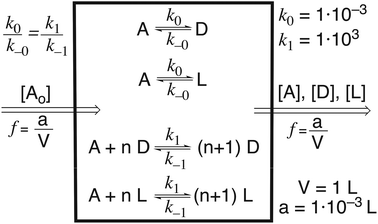Scheme 1 Open system model used in the simulations reported here corresponding to a continuous open flow reactor assuming instant and perfect diffusion of the all species in solution. The reaction rate constants were fixed to k0 = 1 × 10−3, k−0 = 1 × 10−7, k1 = 1 × 103 and k−1 = 1 × 10−4, the chemical mass entry of resources [A0] = 1 × 10−1 mol L−1 and the flow entry/exit rate constant f = 1 × 10−3. f is the ratio between volume of solution entry/exit in the reactor and the reactor volume V that corresponds to reaction rate constants of the pseudo-reactions or matter exchange flows with the surroundings. The results reported here refer to the range 0.1 ≤ n ≤ 2.0 (and in steps of Δn = 0.1).

The set of ordinary differential equations describing the reaction network [(2) + (3) + pseudo-reactions representing the entry and exit of A and the exit of D and L from the reactor] is: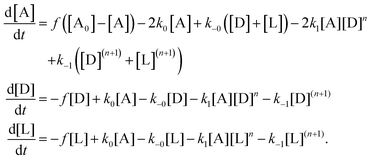(4)

The first term of each differential equation represents the exchange of species with the surroundings: zero order for the entry of A and first order for the exit of A, D and L, where f is the volume exchange, i.e., it is the effective rate constant for these exchanges.

### Simulations and evaluation of thermodynamic parameters

Numerical evaluations were performed (the reaction parameters represented in Scheme 1) using the Mathematica® (12.0.0.) package.43 Mathematica® gives numerical calculations far beyond the machine working precision: starting with numerical parameters with ≥100 decimal points precision and using high working precision methods for the integration routines, it is even possible to reproduce unstable NESS's and to evaluate how these states evolve under very low compositional fluctuations. Such unstable NESS's cannot be simulated using kinetics calculation packages implemented on common computers, whose precision is limited by the machine precision limit.38

The NESS's were evaluated from: (a) the high precision numerical agreement between the dynamical simulations of the ordinary differential equations set (4) (using the NDSolve function) and the static evaluation at the stationary state conditions (using the FindRoot function); (b) the Jacobian stability analysis of the static root evaluation and of the effect of fluctuations on the dynamic evaluations;44 (c) the evaluation of the thermodynamic parameters, for example entropy currents and their differentials, either by the ordinary differential equation set or by SNA, were always found to be in exact numerical agreement. Additional numerical calculations from changing the reaction parameters of Scheme 1 were also carried out in order to confirm the results. Entropy production and entropy exchange calculations were performed through the product of the absolute reaction rate and the corresponding affinity value, using the relative chemical potentials.35,45–47

The entropy production per unit volume V (P) for reaction j is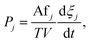(5)
where Afj is the affinity and ξj the extent of the jth reaction. For example, for the enantiomeric D reaction of (2), when expressed, by forward (+) and backward (−) reactions and expressed by the relative chemical potentials (thermodynamic equilibrium concentrations are denoted [Xeq]). For simplification, the temperature term in (5) is suppressed, therefore the P's expressed in the text corresponds in fact to the product TP (being T constant),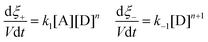(6)(7)
 Af− = −Af+ (8)
 Pint = (k1[A][D]n)Af+ + (k−1[D]n+1)Af− = RT(k1[A][D]n − k−1[D]n+1)ln{k1[A]/k−1[D]} ≥ 0. (9)
Note, that taking into account as above the relative chemical potentials, the evaluation of the pseudo-reactions, corresponding to the matter exchange of the open system (Scheme 1), is straightforward45,47,48 and for example, in the case of species A, yields
 P→A = fA0RTln{[Aeq]/[A]}, (10)
 PA→ = f[A]RTln{[A]/[Aeq]}. (11)
The entropy production (Pint) and entropy flow (Pexch) of the system are then calculated form the sum of the corresponding individual transformations of (4) or in the case of SNA, from its extreme currents’ partial contributions [see Appendices].

The affinities associated to the corresponding reaction and pseudo-reaction sets give the changes of the Helmholtz free energy (model working at constant T and V) with respect to chemical potentials at the thermodynamic equilibrium. The free energy changes associated to the reaction network (called here ΔΔFint) and that associated to the matter exchange with the surroundings (called here ΔFexch) were calculated by the sum of affinities of selecting the corresponding terms of the ordinary differential equation set (4). The SNA [(31) see Appendices] analysis of the thermodynamic forces, because the extreme currents of SNA are combinations of reactions and pseudo-reactions, cannot yield ΔΔFint, but the chemical free energy of the matter transfer that, is identical to ΔFexch. It is remarkable that when the reactor allows for the entry and exit of all species of the reaction network, i.e. when the boundary is permeable to the matter exchange of all species which are in the surroundings at fixed concentrations, then ΔΔFexch = 0 must be zero, but despite this, the entropy exchange (Pexch) is not zero. This because the entropy current results from the addition of the products of the individual forces by the matter exchange rates, and these and their sum will be different from zero.

In summary, the changes in the free-energy state function: the internal (difference with respect to thermodynamic equilibrium: ΔΔFint), that due to the matter exchange (ΔFexch) and the total (ΔΔFtotal = ΔΔFint + ΔFexch), were obtained from the sum of the corresponding affinity differences, as for example in the case of the enantiomer D in reactions (2) and (3).

 ΔΔFint,A⇌D = −RTln{(k0/k−0)([A]/[D]}) − (−RTln{k0/k−0}), (12)
and for reactor exit of D,
 ΔFexch,D→ = AfD = −RTln({[D]/[Deq]}), (13)
We want to stress here, in respect to the discussion below on the energy relationships in the system that the force (Af) in the product leading to the entropy currents, includes too the entropic terms implicit in the chemical potentials corresponding to the different S0 between different chemical species and to the configurational entropy resulting from the chemical transformation. This last play an important role in free energy differences between racemic, scalemic and homochiral mixtures (for the same chemical mass different entropy of mixing depending on their molar fractions). The use of only internal energies to evaluate the energy conservation and the relationship between energy state functions and entropy currents cannot be done when the system shows transformations between different chemical species, that is in contrast with pure physical scenarios.

The term Rate used in Results and discussion corresponds to the rate of the unreacted species A:

 Rate = f[A0] − f([D] + [L]). (14)
Lower Rate values correspond to higher yields of chiral matter. The use of the difference (14) instead of ([D] + [L]) to express the synthetic efficiency of the system was a consequence of the graphical difficulty to visualize small value differences in very small absolute values.

## Results and discussion

### Stability and types of NESS vs. autocatalytic order (n)

The non-catalytic direct reaction (3) dominates the dynamics over the autocatalysis (2) for values between 0 ≤ n < 0.1, but starting at n ≥ 0.1, the autocatalytic dynamics dominates. For n < 1.3 the unique NESS is the stable racemate (Fig. 1; blue squares). Between 1.3 ≤ n ≤ 1.6 in addition to the stable racemic NESS, there are two enantiomeric pairs of scalemic NESS's, one unstable (green dots) and the other is stable (red dots). These two pairs (stable and unstable) have large ee values, with the stable one having ee values closer to the homochiral value (see ESI). At n ≥ 1.7 the pair of unstable scalemic NESS's disappears, and the racemic NESS becomes unstable. This means that the racemic thermodynamic branch is stable for all n ≤ 1.6, that unstable scalemic NESS are possible for 1.3 ≤ n ≤ 1.6, and that SMSB emerges for n ≥ 1.7.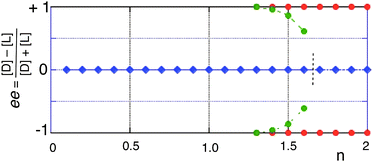Fig. 1 Enantiomeric excess (ee) values corresponding to the NESS's of the system of Scheme 1, as a function of autocatalytic order n. Blue squares, racemic, green dots, unstable scalemic; red dots, stable scalemic. Stable NESS's, indicated by solid lines; unstable NESS's, dashed lines. The racemic NESS's become unstable for 1.6 < n < 1.7 (dashed line). The stable scalemic NESS's have ee > 0.996. The unstable scalemic NESS's show always lower ee values than those of the corresponding stable scalemic NESS's, for example, at n = 1.3, ee = 0.99953 and ee = 0.99569, respectively (see table in ESI†). The stability of each NESS was inferred by Jacobian linear stability analysis of the roots of the right-hand sides of the rate differential equation set and corroborated by numerical evaluation of the dynamics generated by very small fluctuations around the NESS composition.

Dynamic simulations show that within the n range where stable racemic and both unstable and stable scalemic NESS's occur simultaneously (1.3 ≤ n ≤ 1.6), the obtention of either the racemic or the stable scalemic NESS depends on the initial conditions: the stable scalemic NESS can only be obtained when starting from very high ee values, mostly higher than that of the final NESS, but there is a narrow range of high initial ee values yielding small chiral amplifications towards the stable scalemic NESS. Compositional fluctuations at the unstable scalemic NESS (green) determine the evolution towards either the racemic NESS (blue) or the scalemic one (for a detailed discussion see below). In the SMSB region (n ≥ 1.7), any initial scalemic composition or chiral fluctuations at the racemic unstable NESS, even those below the level of statistical chiral noise,49 yields the stable scalemic NESS of the same chiral sign as that of the initial fluctuation. In other words, in the range 1.3 ≤ n ≤ 1.6, the system is tri-stable, in that the unstable scalemic NESS forms a mirror symmetric pair of unstable bifurcation branches (see Fig. 1), whereas for n ≥ 1.7, the system is singly bistable, where the unstable racemic is now the unique single bifurcation branch to two stable mirror symmetric scalemic NESS.

### Entropy currents at the NESS's

We verify that all NESS's obey entropy balance (Pint = −Pexch) and to the numerical precision of the calculations (see Methodology). The entropy production (Pint > 0) vs. the autocatalytic order increases with n and according to the following sequence: racemic NESS > unstable scalemic NESS > stable scalemic NESS (see Fig. 2). The racemic curve in Fig. 2 does not correspond to an exponential growth, it shows negative deviation for n > 0.8. The destabilization of the racemic thermodynamic branch (SMSB) occurs at the critical value of Pint ≈ (1/T) 6.6 × 10−4 (J mol−1 L−1 s−1). We point out that for the range 1.3 ≤ n ≤ 1.6, and if the system starts off at any one of the unstable scalemic NESS, then the entropy production can either increase to a maximum value corresponding to the stable racemic NESS or can decrease to a minimum value corresponding to a stable scalemic NESS. Therefore, in this range then, the model would appear to satisfy the so-called principles of either the minimum or maximum entropy production (MaxEPP,50 MinEPP22) depending exclusively on the sign of the fluctuation about the unstable scalemic. This means that both “principles” are in contradiction here, as which of the two will be operative depends crucially on the sign of the fluctuation about the unstable scalemic NESS. However, in the case of the solidly proven MinEPP, disagreement is to be expected since our system is well beyond the linear regime, where the MinEPP principle is valid.17,18,20 For n > 1.7, the racemic NESS becomes unstable and the system can only minimize the entropy production (see below) by ending up in the stable scalemic NESS.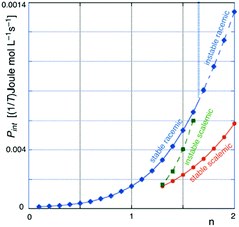Fig. 2 Entropy production (Pint = −Pexch ≥ 0) of the possible NESS's (open flow system and the same reaction parameters as in Scheme 1) for different n values (full line = stable NESS; dashed line = unstable NESS). The scalemic NESS corresponds in fact to the degenerate pair of enantiomeric states. At a Pint (at 300 K) ≈ 2 × 10−6 J mol−1 L−1 s−1 (1.6 < n < 1.7), the racemic NESS becomes unstable leading to SMSB.

It is worth noting that all the three curves of Fig. 2 show nearly exponential correlation with order n for low autocatalytic orders, negative deviations appear when n increases. Furthermore, with respect to the efficiency towards species selection, it is surely significant that, for the thermodynamic branch, the exponential dependence on n is lost for n ≥ 1.

### Entropy balance as a necessary but not sufficient condition for existence of NESS

The evaluation of all the states where P = 0 = Pint + Pexch shows that this constraint is a necessary, but not sufficient condition for a NESS: Fig. 3 shows, the P = 0 contours, for a range of n, at constant total chemical mass, depending on [A] and on the enantiomeric fraction fr (see Fig. 3 for its definition). The stationary states fulfilling the constraint form curve lines at the P = 0 surface where the NESS's are only specific points within these curves. It is worth noting, that these P = 0 curves, in addition to the local equilibrium assumption, include the constraint of the balance of entropy currents: a stronger condition than that of local equilibrium. This means that the curve lines of Fig. 3 represent singular pathways in the compositional space, between specific states, where the transformation between states is possible under the constraint of the entropy flow balance. Finally, in contrast with what could be expected in reversible thermodynamics, the entropy current balance constraint, Pint = −Pexch, does not coincide with a Pint minimum: only at the state showing thermodynamic equilibrium composition is Pint an extremum and obviously equal to zero.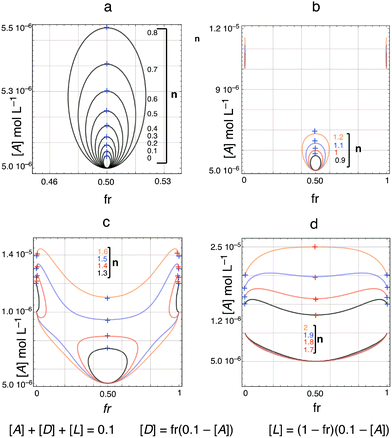Fig. 3 Curves representing the condition of stationary entropy balance (P = 0 = Pint + Pexch) for the system of Scheme 1 plotted as functions of [A] (indirect expression of the internal free energy change) and of the bias from the racemic composition (fr = 0.50 for the racemate and fr = 0 or 1 for the homochiral composition) for different autocatalytic orders n. The NESS are specific points as marked within these curve lines: stable NESS: +blue; unstable NESS's: +red.

At autocatalytic orders n where the racemate is the unique stable NESS (Fig. 3a and b), the curves in the P = 0 surface form an ellipsoid where one apex corresponds to a non-equilibrium state showing the same composition as that of thermodynamic equilibrium (lowest [A] value), and the other apex corresponds to the stable racemic NESS (highest [A] value). When the autocatalytic order n increases, the ellipsoid becomes larger, elongating towards larger [A] values, and becoming wider in fr. It is worth noting that the P = 0 curve lines between these two racemic states pass through (scalemic) states showing ee values different from zero. This means that for the transformation in the open system, from initial conditions having the thermodynamic equilibrium composition, the way from the initial equilibrium racemate to the final non-equilibrium stable racemate, as well as in the thermodynamic limit, the “full reversible” pathway in the open system passes through non-racemic states, before the slower racemization pathway is shown, the mixture: the transitory ee ≠ 0 increases when the autocatalytic order n increases, such as could be expected in stochastic kinetics for the closed system, due to the statistical character of the racemate composition.49

About n = 1 there are states of ee values near to homochirality which are not stationary states (Fig. 3b). However, at n ≥ 1.3 scalemic NESS's emerge there: one enantiomeric pair of unstable scalemic NESS and one enantiomeric pair of stable scalemic NESS (Fig. 3c), and very close together. The unstable NESS pair has lower ee values that the stable pair. Furthermore, at n = 1.5, the central ellipsoid merges with the scalemic curves separating into two non-connected curve lines: one containing the state of thermodynamic equilibrium composition, that do not have any NESS and represents the “full reversible” deracemization of the corresponding non-stationary racemic state. The other curve line at higher [A] (higher affinity) contains all the NESS of the system. Increase of the autocatalytic order moves the NESS curve towards higher affinity values and changes the curvatures of the line. In the range 1.3 ≤ n ≤ 1.6 the thermodynamic branch (racemic NESS's) remains stable despite the emergence of pairs of stable scalemic NESS's. Such as discussed above, in this range of autocatalytic order, the stable scalemic NESS's correspond to final states which can only be obtained when starting from initial conditions showing very high ee values.

### Energy conservation relationships at the NESS

The essence of irreversible thermodynamics is the relationship between energy state functions and the physical potential as determined by the entropy currents of the system. Energy conservation in open systems was subjected to an historical debate which is settled nowadays, once the dissipated heat/energy for sustaining the chemical work inside the system and to sustain the reaction network away from thermodynamic equilibrium is defined (see e.g.ref. 51–54). The inclusion of matter exchange flows in the set of ordinary differential equations, and especially in the SNA approach, allows to obtain a description of the relationship between the different energy contributions when bi-stability phenomenon occurs.

The first principle (energy conservation) can be expressed at the NESS in our homogeneous system with isothermal matter and energy exchange by

 ΔΔFint + ΔFexch = Work + Q. (15)
In our case, since T and V are constant, there is no generation of work performed on the surroundings (Work = 0). Therefore, Q is the heat/energy that cannot be used for work, and expressed in entropic term is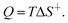(16)
Eqn (15), instead of the commonly employed internal energies, uses free energy differences with respect to the thermodynamic equilibrium: ΔFint = (ΔFint − ΔF0int), because different chemical species have different S0 values and mixtures of different compositions (different molar fractions) also have different configurational entropies (entropy of mixing). ΔΔFint is easily calculated from the sum of the affinities of all reactions. Note that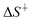is an entropic term different from those included in the free energy differences on the left-hand side of (15).55 ΔFexch represents the energy change generated in the matter transfer with the surroundings, and corresponds to the sum of the pseudo-reaction forces of the entry flow of species i and the exit flow of species j. These forces can be expressed by the relative chemical potentials as follows: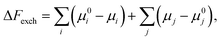(17)
which are the forces used in the eqn (10) and (11) expressed as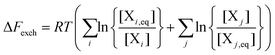(18)
It is worth noting that any species that both enters and exits the Scheme 1 flow reactor, yields a zero contribution to the ΔFexch term, but leads to a finite value to the entropy flow (10) and (11). This is because the latter results from the product of force by a current.can separated in the internal and exchange contributions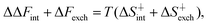(19)
where equality is inferred when assuming the first principle.

The combination of first and second principles leads (15) to the time dependent expression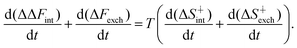(20)
The two terms at the right of (20) correspond respectively to Pint and Pexch and can be calculated, in contrast to, by the product of the free energy terms, at the left of eqn (15), by their absolute rates. At a NESS, the species concentrations are constant, so the left-hand side terms of (20) are zero and hence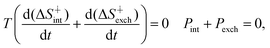(21)
and corresponds to the entropy currents balance of equation [Pint + Pexch = 0], where Pint = −Pexch(5) at any NESS and Pint = 0 = Pexch at thermodynamic equilibrium (see Appendices).

### Free energies and productive rates at the NESS's

Fig. 4 displays the effect of the increase of the autocatalytic flow due to the order n of the autocatalysis. The relative ordering of the free energy differences between NESS (see Fig. 4) vs. the autocatalytic order n is opposite to the relative ordering of the entropy productions (Fig. 2): here by increasing n, the free energy difference goes as: racemic NESS < unstable scalemic NESS < stable scalemic NESS. This pattern holds for the internal, the exchange and total free energy differences. The stable scalemic NESS's show, apparently, a linear relationship between ΔΔFint and the autocatalytic order n. The racemic thermodynamic branch, for stable as well unstable NESS's, shows an exponential growth of ΔΔFexch.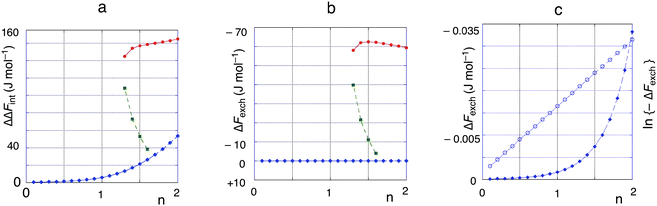Fig. 4 Free energy changes of the NESS at different autocatalytic orders (n). Blue dots, racemic NESS's (stable for n ≤ 1.6, unstable for n ≥ 1.7); green dots, unstable scalemic NESS's; red dots, stable scalemic NESS's. Fig. 4c is an amplification of the racemic NESS curve of Fig. 4b showing the exponential increase of the external free energy change with the autocatalytic order n.

Fig. 5 shows how the constraint Pint = −Pexch at the NESS corresponds to the inequality ΔΔFint > −ΔFexch where ΔΔFint > 0 and ΔFexch ≤ 0. This inequality, expressed as a ratio, is orders of magnitude higher for the racemic NESS's than for the scalemic NESS's, and larger for the unstable scalemic NESS than for the stable scalemic NESS. ΔΔFint increases with the autocatalytic order n for all the racemic NESS's and the stable scalemic NESS, but decreases for the unstable scalemic NESS's. ΔFexch increases (in absolute value) with the autocatalytic order n for all NESS's of the racemic thermodynamic branch and decreases for the unstable scalemic NESS's. However, the stable scalemic NESS's show an initial increase of ΔFexch, (in absolute value) but for n > 1.5, the sign of the slope changes.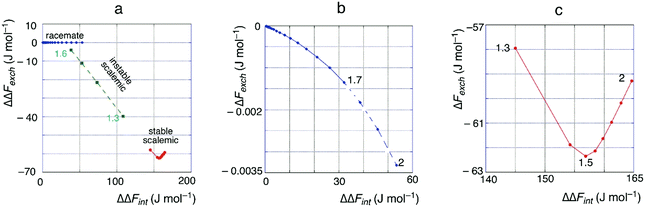Fig. 5 Relationships between ΔΔFexch/ΔFint leading to the entropy balance at NESS's. Fig. 5b and c are amplifications of the racemate and the stable scalemic curves, respectively, of Fig. 5a. The graphics show how the increase of the forces, both internal and at the boundary, act in in order to change the matter flows and so achieve the entropy balance (Pint + Pexch = 0) at the NESS's.

Fig. 6 shows how, in contrast with what is expected in reversible thermodynamics, the production of chiral matter decreases, in spite of significant increases in ΔΔFint.§ The extrapolation of the evolution of the curve of the unstable scalemic NESS's (Fig. 6b and c) shows the intersection with the racemic thermodynamic branch at the critical point of the SMSB bifurcation when the thermodynamic branch becomes unstable.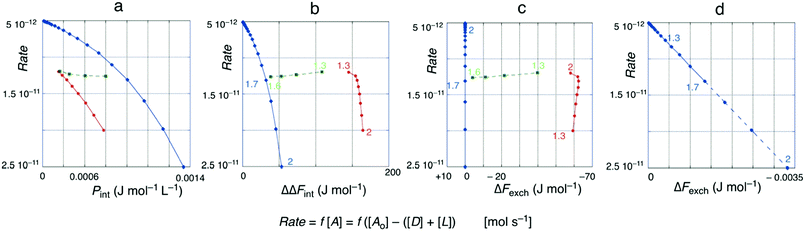Fig. 6 (a) Dependence (decrease) of the productive rate (note inverted ordinate axis of the rate ≡ unproductive rate) with respect to the entropy production. (b)–(d) Relationship between free energy changes and productive rate. Fig. 6d is the amplification of the racemic NESS's of Fig. 6c. The productive rate is expressed (mol s−1) through f [A], i.e. the concentration of unreacted A.

Note, that the relative ordering of the type of NESS's is the inverse when we compare the rate either with the entropy production or the forces (compare Fig. 6a with Fig. 6b).

The linearity of the Rate vs. ΔΔFexch representation for the thermodynamic branch (Fig. 6d) is consequence of: (a) the matter exchange rate at the NESS is the total transformation from A to D and L performed by the system, i.e. the Rate value, and the Af of this “total” synthesis is identical to ΔFexch; (b) for the thermodynamic branch ΔFexchRT, so that its relationship with Rate is linear, despite the kinetic non-linearity, as given by the first-order Taylor expansion of its exponential relationship (see e.g. in ref. 17 p. 371 and ref. 53). Note that the Af value corresponding to the total reaction of the system is ΔFexch: for example a system showing the entry and the exit of all chemical species of the reactor create NESS's that show ΔFexch = 0 and no net productive rate, as it is the case of the called non-productive cycles in the description of biochemistry networks.

### Relationships between Pint, affinities (free energy changes) and reaction rates

The relationships made explicit in Fig. 5 and 6 are the result of the fine tuning between the forces and the absolute rates, both for the internal reaction network as well as for the matter exchange, that operate automatically in order to fulfil the thermodynamic constraint (21). The increase of the autocatalytic order n, leads to the decrease of the chemical production rate thanks to the fact that more unreacted species A can exit the open flow reactor, and even though ΔΔFint increases for the stable NESS's.

The thermodynamic branch reveals a linear relationship ΔΔFint/Pint (see Fig. 7) suggesting that for the racemic NESS, there is an exact differential relationship between Pint and Afint (see Chapter IX in ref. 22). In the graphical representation of Pint (−Pexch) with respect to ΔΔFint, to ΔFexch or to ΔΔFtotal (ΔΔFtotal = ΔΔFint + ΔFexch) (Fig. 7 and ESI), the most relevant trend is the relationship Pintvs. ΔΔFint. The slope ΔΔFint/Pint is negative for the unstable scalemic NESS, but positive for the thermodynamic branch and for the stable scalemic NESS's.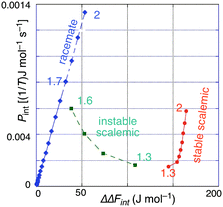Fig. 7 Relationship between ΔΔFint and Pint. Blue dots, racemic NESS's (stable for n ≤ 1.6, unstable for n ≥ 1.7); green dots, unstable scalemic NESS's; red dots, stable scalemic NESS's. The relationship of ΔΔFint and ΔFint to Pint (=−Pexch) is linear for the racemic NESS (thermodynamic branch), and is probably also linear for the stable scalemic NESS's within the SMSB region (n ≥ 1.7).

Furthermore, the slope in the SMSB range of n (n ≥ 1.7) is slightly smaller for the stable scalemic NESS's than for the unstable racemic NESS. However, when ΔΔFexch is taken into account, i.e. using the sum of both energies (ΔΔFtotal) the results show only an approximate linearity (see Fig. S1, ESI). However, the effect that the forces, represented by ΔΔFtotal, have on the entropy production Pint and on the system evolution, is accurately determined by the differential expression, dFP/dt ≤ 0 of the GEC (see Appendices), as we discuss in the following section.

### General evolution criterion (GEC) and stability at the NESS's

According to the GEC, the evolution of any state is determined by the decrease in the rate of change of P, i.e. the sum of the entropy production and the entropy flow, with respect to the temporal derivative of the forces/affinities dFP/dt ≤ 0 (with equality holding at any NESS, as well as for thermodynamic equilibrium). Notice that this condition is fulfilled in the entire range of extended nonlinear thermodynamics, while for the linear regime, one has dFP/dt = dJP/dt. In reversible thermodynamics, concerning isolated systems, the terms do not include any interaction with the surroundings.

The numerical evaluation of dFP/dt, for the transformations discussed above, fulfils the GEC in the evolution.

The numerical simulations of the effect of a fluctuation the evolution from an initial NESS towards a final NESS, and with respect to the GEC, are summarized as follows.

Stable racemic and scalemic NESS's. All fluctuations around a stable NESS lead to the return to that initial NESS. This describes stable NESS's as states situated in a potential well described by −dFP/dt. Any fluctuation changing either the affinity values and/or the ee values leads to the return to the stable initial NESS. Therefore, the stable NESS's are attractors. For example, the racemic NESS, which is the unique stable NESS for 0 < n < 1.3, is an attractor for any initial composition. In the autocatalytic range of n where both stable racemic and stable scalemic NESS's coexist, both are attractors (i.e., the system is bistable). In this respect, the racemic NESS is an attractor for a wide range of initial compositions and the scalemic NESS is an attractor for initial compositions having large ee values, i.e. both NESS act as attractors for specific domains of composition space.
Unstable NESS's. Here, the fluctuation leads to negative dFP/dt values which dynamically evolve to zero, but towards a different stable NESS, instead of returning to the initial unstable one. However, there is a difference between the behaviour starting from the unstable racemic NESS's and that starting from the unstable scalemic NESS's.
Unstable racemic NESS (n ≥ 1.7). Fluctuations about the NESS that retain the ideal racemic composition lead to the return to the unstable racemic NESS. In fact, any initial conditions showing the ideal racemic composition lead back to the unstable racemic NESS. This is a proof of the computational methodology used to avoid the machine-generated computational noise, but the significant point is that the unstable racemic NESS, in the absence of racemic composition fluctuations, is an attractor for any racemic composition. Nevertheless, this does not change the actual unstable character of these racemic NESS's, because stochastic symmetrical fluctuations in the reactions between A and the enantiomers (D and L) which maintain the racemic composition, are unlikely compared those leading to biases away from the racemic and racemic fluctuations. Therefore, even when starting from the ideal racemic composition, in a real system some fluctuation departing from ee = 0 will occur, and the system will end up at a stable scalemic NESS having the same chiral sign as that of the initial chiral fluctuation.
Unstable scalemic NESS (1.3 ≤ n ≤ 1.6). Any compositional fluctuation leads to an evolution either towards the stable racemic NESS or towards the stable scalemic NESS. In this range, and because of the chirality/enantiomerism phenomenon, the system is tristable. It is remarkable that the evolution towards one of the two attractors does not necessarily agree with an “intuitive” prediction based on expectations of reversible thermodynamics: a fluctuation about the unstable scalemic NESS that decreases the ee value does not necessary evolve towards the stable racemic NESS and a fluctuation increasing the ee value does not necessary evolve towards the corresponding stable scalemic NESS. In fact, there is a small range of ee values, smaller than that of unstable scalemic NESS, that evolves towards the stable scalemic NESS.

Fig. 8 gives an example of the relationship between the fluctuation and dFP/dt and how the dynamic evolution is always such that dFP/dt = 0 at the final NESS, which can either coincide with the initial NESS, if this is stable, or to a stable NESS if the initial NESS was unstable.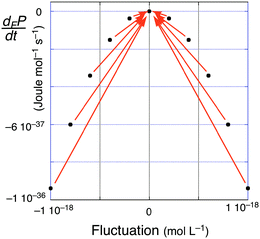Fig. 8 Example of the simulation of the effect of a fluctuation in the composition of one species at a NESS on dFP/dt. The arrows indicate the dynamic evolution in the dFP/dt value towards a final stable NESS. This can either be the same NESS, in the case of an initially stable NESS, or a different stable NESS in the case of starting off from an unstable NESS.

Note that a representation, similar to the chemical profiles of the reaction activation energies versus the reaction coordinate, should not be used in the case of dissipative systems. Coordinate reaction profiles arise from molecular considerations of the reaction pathway, and assume micro-reversibility, i.e. the reaction must follow the same pathway in the forward and reverse directions. Here we apply the micro-reversibility constraint to the system reaction parameters in order to obtain a correct modelling of the enantiomerism phenomenon. However, hypersurfaces representing the behaviour dFP/dt ≤ 0 are graphical attractors displays of the whole system, typical in Lotka–Volterra type graphics (see e.g.ref. 56), where the system evolution at each point can occur only in those directions in strict compliance with dFP/dt < 0 (GEC).

The system analysis here has, for each point in composition space, a dynamic solution obeying dFP/dt < 0. However, other systems having only unstable NESS would exhibit an oscillatory character, and systems, for a specific composition, having more than one solution yielding dFP/dt < 0, could exhibit an aperiodic chaotic time dependence.

## Conclusions

Enantioselective autocatalysis, in addition to its interest regarding the problem of the origin of biological homochirality, is a paradigm of reaction networks able to give rise to different NESS's. For example, compared to the extensively studied Schlögl model for bistability,57,58 enantioselective autocatalysis shows a range of bistability, but also a double bistable range, that is, tri-stability, with three stable NESS's.

The inclusion of the matter exchange flows as a fundamental part of the overall reaction network, and together with SNA, gives a correct energy analysis of the heat dissipation, non-usable to produce work, in isothermal open systems. In contrast with the widely used clamped approximation, the inclusion of matter flows gives an adequate description of the relationship between entropy currents and energy functions and energy conservation in open systems.

The complete agreement of the GEC with the complexity of the NESS's this the model gives rise to, raises the question of why its use has been so infrequent in extended nonlinear thermodynamics and suggests that it could be useful in simulations for predictions on the systems evolution in the non-linear regime of non-equilibrium thermodynamics.

## Conflicts of interest

There are no conflicts to declare.

## Appendices

### Brief synopsis of non-equilibrium thermodynamics

Entropy flow, between the system and its surroundings, and the internal entropy production in open systems have been defined as ontological categories in irreversible thermodynamics.21,22,59 In this respect, the change in system entropy can be divided in two parts, one corresponding to that taking place inside the system (internal) and the other corresponding to changes arising through the interaction with the surroundings (exchange):
 S = Sint + Sexch, (22)
therefore, the differential can be expressed as
 dS = dSint + dSexch, (23)
and on the temporal scale, we define P (P = dS/dt) so that
 P = Pint + Pexch, (24)
where Pint ≥ 0 is the internal entropy production, and Pexch is the entropy flow or exchange between the system and its surroundings, the latter can be positive or negative.

The entropy production is expressed by the sum of products of forces Xi and the currents Ji that they generate: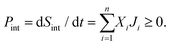(25)
For the case of chemical reactions, the expression for the internal entropy production is straightforward: the force Xi is the affinity and the current Ji is the absolute reaction rate.17,22 At a stationary state dS/dt = 0, we thus have
 P = 0; Pint = −Pexch ≥ 0, (26)
with the equality at zero holding when the stationary state corresponds to thermodynamic equilibrium (isolated systems, i.e., absence of entropy flow, Pexch, with the surroundings).17,18,22 However, in the case of models and simulations of chemical reactions in open systems, the entropy exchange term Pexch is typically suppressed by the so-called “clamped” approximation, which imposes constant fixed concentrations at the boundaries of the system. This leads to errors and artefacts such as stable NESS's. This is a crucial issue in the description of bifurcations leading to NESS's distinct from those lying on the thermodynamic branch, i.e. the description of the SMSB phenomenon, and for the correct interpretation of the General Evolution Criterion (GEC) in the non-linear thermodynamic regime.

The entropy production and entropy flow (Pint, Pexch) play the role of driving potential in irreversible thermodynamics. In the linear regime, it becomes a physical potential, in much the same way that the Helmholtz or the Gibbs free energy are in equilibrium thermodynamics, despite the fact that P is not a thermodynamic state function.20 The total time derivative can be split into two contributions: one with respect to the forces (F) and the other with respect to the currents (J),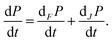(27)
In the linear regime (Theorem of Minimum Entropy Production) the role of P as a physical potential is clear, because one has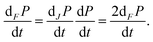(28)
However, in the non-linear regime, the role of P in the system evolution is indirect, because dFP/dt ≠ dJP/dt, but the general evolution criterion (GEC) establishes that the forces evolve in such a way so as to minimize the entropy production: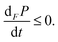(29)
In the non-linear regime, the differential of P with respect to the temporal derivative of the currents J can be either positive or negative (see ref. 22 Chapter IX), but it is (29), the derivative of P with respect to the temporal derivative of the forces, that was proposed to determine the stability/evolution of a state.21,22 The GEC is rarely if ever mentioned nowadays in reports of far from equilibrium chemical systems, despite being the fundamental link between reversible and irreversible thermodynamics, arising as an algebraic consequence of the correspondence of signs between force/affinities (Af), and their current effects/absolute rates (to be published elsewhere).

### Stoichiometric network analysis

Stoichiometric network analysis (SNA) is a powerful tool for describing reaction networks.12,53,60 SNA has been applied recently to the simulation of spontaneous mirror symmetry breaking (SMSB) in enantioselective autocatalytic networks.29 The description of the so-called elementary flux modes (EFM) or extreme currents, that is, the irreducible set of combinations of the reaction flows, may be composed simultaneously by the chemical reactions and the pseudo-reactions. This means that SNA defines the system as a whole, (i.e., reactions and flows) and the partition of the entropy currents among these extreme flows gives a unique description of how the coupling between the reactions determines the physical/chemical behaviour of the chemical network.35,45

The reaction network in Scheme 1 is defined by the following set of individual reactions, which make up the stoichiometric matrix for SNA:

 A → D A → L

 D → A L → A

 A + nD → (n + 1)D A + nL → (n + 1)L

 (n + 1)D → A + nD (n + 1)L → A + nL

 → A

 A →

 D →
 L →. (30)
The thirteen elementary or extreme currents (Ei) for (9), as determined by the COPASI program package,61 are as follows:
 E1: (1/3); A → L (1/2); L → A

 E2: (1/2); L → A (1/3); A + nL → (n + 1)L

 E3: (1/3); A → L (1/2); (n + 1)L → nL + A

 E4: (1/3); A + nL → (n + 1) L (1/2); (n + 1) L → nL + A

 E5: (1/3); A → D; (1/2); D → A

 E6: (1/2); D → A; (1/3); A + nD → (n + 1)D

 E7: (1/3); A → D; (1/2); (n + 1) D → nD + A

 E8: (1/3); A + nD → (n + 1)D; (1/2); (n + 1)D → nD + A

 E9: (1/5); → A A →

 E10 (1/5); → A (1/3); A → L (1/2); L →

 E11: (1/5); → A (1/3); A + nL → (n + 1)L; (1/2); L →

 E12: (1/5); → A (1/3); A → D (1/2); D →
 E13: (1/5); → A; (1/3); A + nD → (n + 1)D; (1/2)D. (31)

The corresponding fractional coefficients are needed for the evaluation of the affinity contribution of each reaction to each extreme current, and for the calculation of the partial entropy currents. For more details regarding the SNA method used here, see ref. 35, 44 and 45.

## Acknowledgements

The authors acknowledge the coordinated research grants CTQ2017-87864-C2-1(2)-P(MINECO).

## Notes and references

1. G. Lente, Homogeneous chiral autocatalysis: A simple, purely stochastic kinetic model, J. Phys. Chem. A, 2004, 108, 9475–9478 CrossRef CAS.
2. G. Lente, Stochastic Kinetic Models of Chiral Autocatalysis: A General Tool for the Quantitative Interpretation of Total Asymmetric Synthesis, J. Phys. Chem. A, 2005, 109, 11058–11063 CrossRef CAS PubMed.
3. D. K. Kondepudi and K. Asakura, Chiral Autocatalysis, Spontaneous Symmetry Breaking, and Stochastic Behavior, Acc. Chem. Res., 2001, 34, 946–954 CrossRef CAS PubMed.
4. R. Plasson, D. K. Kondepudi, H. Bersini, A. Commeyras and K. Asakura, Emergence of homochirality in far-from-equilibrium systems: Mechanisms and role in prebiotic chemistry, Chirality, 2007, 19, 589–600 CrossRef CAS PubMed.
5. D. G. Blackmond, Asymmetric autocatalysis and its implications for the origin of homochirality, Proc. Natl. Acad. Sci. U. S. A., 2004, 101, 5732–5736 CrossRef CAS PubMed.
6. J. M. Ribó and D. Hochberg, Chemical basis of biological homochirality during the abiotic evolution stages on earth, Symmetry, 2019, 11, 814 CrossRef.
7. S. A. Kauffman, Autocatalytic sets of proteins, J. Theor. Biol., 1986, 119, 1–24 CrossRef CAS PubMed.
8. M. Eigen, J. McCaskill and P. Schuster, Molecular quasi-species, J. Phys. Chem., 1988, 92, 6881–6891 CrossRef CAS.
9. W. Hordijk, J. Hein and M. Steel, Autocatalytic sets and the origin of life, Entropy, 2010, 12, 1733–1742 CrossRef CAS.
10. A. J. Bissette and S. P. Fletcher, Mechanisms of Autocatalysis, Angew. Chem., Int. Ed., 2013, 52, 12800–12826 CrossRef CAS PubMed.
11. L. von Bertalanffy, The Theory of Open Systems in Physics and Biology, Science, 1950, 111, 23–29 CrossRef CAS PubMed.
12. R. Heinrich and S. Schuster, The Regulation of Cellular Systems, Springer, US, 1996 Search PubMed.
13. J. M. Ribó, D. Hochberg, J. Crusats, Z. El-Hachemi and A. Moyano, Spontaneous mirror symmetry breaking and origin of biological homochirality, J. R. Soc., Interface, 2017, 14, 20170699 CrossRef PubMed.
14. J. M. Ribó, C. Blanco, J. Crusats, Z. El-Hachemi, D. Hochberg and A. Moyano, Absolute asymmetric synthesis in enantioselective autocatalytic reaction networks: Theoretical games, speculations on chemical evolution and perhaps a synthetic option, Chem. – Eur. J., 2014, 20, 17250–17271 CrossRef PubMed.
15. J. Crusats, D. Hochberg, A. Moyano and J. M. Ribó, Frank model and spontaneous emergence of chirality in closed systems, ChemPhysChem, 2009, 10, 2123–2131 CrossRef CAS PubMed.
16. A. Eschenmoser, The search for the chemistry of life’s origin, Tetrahedron, 2007, 63, 12821–12844 CrossRef CAS.
17. D. Kondepudi and I. Prigogine, Modern Thermodynamics: From Heat Engines to Dissipative Structures: Second Edition, 2014 Search PubMed.
18. S. R. de Groot and P. Mazur, Non-Equilibrium Thermodynamics, North-Holland, Amsterdam, 1962 Search PubMed.
19. P. Glansdorff and I. Prigogine, On a general evolution criterion in macroscopic physics, Physica, 1964, 30, 351–374 CrossRef.
20. P. Glansdorf and I. Prigogine, Thermodynamic theory of structure, stbility and fluctuations, Wiley-Interscience, London, 1971 Search PubMed.
21. G. Nicolis and I. Prigogine, Self-organization in non-equlibrium systems, Wiley-Interscience, New York, 1977 Search PubMed.
22. P. Glansdorff and I. Prigogine, Thermodynamic theory of structure, stbility and fluctuations, Wiley-Interscience, London, 1971 Search PubMed.
23. P. Glansdorff, The general evolution criterion, in Thermodynamics in contemporary dynamics, ed. P. Glansdorff, Springer, Vienna, 1971, pp. 29–34 Search PubMed.
24. E. Szathmáry, The origin of replicators and reproducers, Philos. Trans. R. Soc., B, 2006, 361, 1761–1776 CrossRef PubMed.
25. C. Fernando, G. Von Kiedrowski and E. Szathmáry, A Stochastic Model of Nonenzymatic Nucleic Acid Replication: “Elongators” Sequester Replicators, J. Mol. Evol., 2007, 64, 572–585 CrossRef CAS PubMed.
26. P. Schuster and K. Sigmund, Replicator dynamics, J. Theor. Biol., 1983, 100, 533–538 CrossRef.
27. R. Wegscheider, Ueber simultane Gleichgewichte und die Beziehung zwische Thermodynamik and Reaktionskinetic homogener Systeme, Z. Phys. Chem., 1902, 39, 257–302 Search PubMed.
28. D. G. Blackmond and O. K. Matar, Re-examination of reversibility in reaction models for the spontaneous emergence of homochirality, J. Phys. Chem. B, 2008, 112, 5098–5104 CrossRef CAS PubMed.
29. M. Stich, J. M. Ribó, D. G. Blackmond and D. Hochberg, Necessary conditions for the emergence of homochirality via autocatalytic self-replication, J. Chem. Phys., 2016, 145, 074111 CrossRef PubMed.
30. S. Lifson and H. Lifson, A Model of Prebiotic Replication: Survival of the Fittest versus Extinction of the Unfittest, J. Theor. Biol., 1999, 199, 425–433 CrossRef CAS PubMed.
31. R. Plasson, A. Brandenburg, L. Jullien and H. Bersini, Autocatalyses, J. Phys. Chem. A, 2011, 115, 8073–8085 CrossRef CAS PubMed.
32. G. von Kiedrowski, A Self-Replicating Hexadeoxynucleotide, Angew. Chem., Int. Ed. Engl., 1986, 25, 932–935 CrossRef.
33. M. Kindermann, I. Stahl, M. Reimold, W. M. Pankau and G. von Kiedrowski, Systems Chemistry: Kinetic and Computational Analysis of a Nearly Exponential Organic Replicator, Angew. Chem., Int. Ed., 2005, 44, 6750–6755 CrossRef CAS PubMed.
34. J. M. Ribó, J. Crusats, Z. El-Hachemi, A. Moyano and D. Hochberg, Spontaneous mirror symmetry breaking in heterocatalytically coupled enantioselective replicators, Chem. Sci., 2016, 8, 763–769 RSC.
35. D. Hochberg and J. M. Ribó, Entropic Analysis of Mirror Symmetry Breaking in Chiral Hypercycles, Life, 2019, 9, 28 CrossRef CAS PubMed.
36. F. C. Frank, On spontaneous asymmetric synthesis, Biochim. Biophys. Acta, 1953, 11, 459–463 CrossRef CAS.
37. J. R. Islas, D. Lavabre, J.-M. Grevy, R. H. Lamoneda, H. R. Cabrera, J.-C. Micheau and T. Buhse, Mirror-symmetry breaking in the Soai reaction: A kinetic understanding, Proc. Natl. Acad. Sci. U. S. A., 2005, 102, 13743–13748 CrossRef CAS PubMed.
38. D. G. Blackmond, Autocatalytic Models for the Origin of Biological Homochirality, Chem. Rev. DOI:10.1021/acs.chemrev.9b00557.
39. V. Avetisov and V. Goldanskii, Mirror symmetry breaking at the molecular level, Proc. Natl. Acad. Sci. U. S. A., 1996, 93, 11435–11442 CrossRef CAS PubMed.
40. J. M. Ribó, J. Crusats, Z. El-Hachemi, A. Moyano, C. Blanco and D. Hochberg, Spontaneous Mirror Symmetry Breaking in the Limited Enantioselective Autocatalysis Model: Abyssal Hydrothermal Vents as Scenario for the Emergence of Chirality in Prebiotic Chemistry, Astrobiology, 2013, 13, 132–142 CrossRef PubMed.
41. C. Blanco, J. Crusats, Z. El-Hachemi, A. Moyano, D. Hochberg and J. M. Ribõ, Spontaneous emergence of chirality in the limited enantioselectivity model: Autocatalytic cycle driven by an external reagent, ChemPhysChem, 2013, 14, 2432–2440 CrossRef CAS PubMed.
42. R. I. Cukier, C. M. Fortuin, K. E. Shuler, A. G. Petschek and J. H. Schaibly, Study of the sensitivity of coupled reaction systems to uncertainties in rate coefficients. I Theory, J. Chem. Phys., 1973, 59, 3873–3878 CrossRef CAS.
43. Wolfram Research Inc, Mathematica, Wolfram Research, Inc, Chmpaong, Illinois, 12.0., 2019.
44. D. Hochberg, R. D. Bourdon García, J. A. Ágreda Bastidas and J. M. Ribó, Stoichiometric network analysis of spontaneous mirror symmetry breaking in chemical reactions, Phys. Chem. Chem. Phys., 2017, 19, 17618–17636 RSC.
45. D. Hochberg and J. M. Ribó, Stoichiometric network analysis of entropy production in chemical reactions, Phys. Chem. Chem. Phys., 2018, 20, 23726–23739 RSC.
46. D. Kondepudi and L. Kapcha, Entropy production in chiral symmetry breaking transitions, Chirality, 2008, 20, 524–528 CrossRef CAS PubMed.
47. H. Mahara and T. Yamaguchi, Entropy balance in distribute reversible Gray-Scott model, Phys. D, 2010, 239, 729–734 CrossRef CAS.
48. K. Banerjee and K. Bhattacharyya, Does an irreversible chemical cycle support equilibrium?, 2013, arXiv:1309.0960v1 [physics.chem-ph].
49. K. Mislow, Absolute asymmetric synthesis: a commentary, Croat. Chem. Acta, 1996, 69, 485–511 CAS.
50. R. C. Dewar, Maximum entropy production and the fluctuation theorem, J. Phys. A: Math. Gen., 2005, 38, L371–L381 CrossRef.
51. Y.-J. Yang and H. Qian, Unified formalism for entropy production and fluctuation relations, Phys. Rev. E, 2020, 101, 22129 CrossRef PubMed.
52. H. Ge and H. Qian, Physical origins of entropy production, free energy dissipation, and their mathematical representations, Phys. Rev. E: Stat., Nonlinear, Soft Matter Phys., 2010, 81, 051133 CrossRef PubMed.
53. H. Qian, D. A. Beard and S. Liang, Stoichiometric network theory for nonequilibrium biochemical systems, Eur. J. Biochem., 2003, 270, 415–421 CrossRef CAS PubMed.
54. S. I. Serdyukov, Generalization of the evolution criterion in extended irreversible thermodynamics, Phys. Lett. A, 2004, 324, 262–271 CrossRef CAS.
55. F. Schlögl, Produzierte Entropie als statistisches Maß, Z. Phys., 1967, 198, 559–568 CrossRef.
56. J. M. Ribó and D. Hochberg, Competitive exclusion principle in ecology and absolute asymmetric synthesis, Chirality, 2015, 27, 722–727 CrossRef PubMed.
57. F. Schlögl, Chemical reaction models for non-equilibrium phase transitions, Z. Phys., 1972, 253, 147–161 CrossRef.
58. R. G. Endres, Entropy production selects nonequilibrium states in multistable systems, Sci. Rep., 2017, 7, 14437 CrossRef PubMed.
59. H. Wehrt, in Offene Systeme I, ed. E. U. von Weizsäcker, Klen-Clotta, Stuttgart, Germany, 1974, pp. 114–199 Search PubMed.
60. B. L. Clarke, Stability of Complex Reaction Networks, Adv. Chem. Phys., 1980, 43, 1–215 CAS.
61. S. Hoops, S. Sahle, R. Gauges, C. Lee, J. Pahle, N. Simus, M. Singhal, L. Xu, P. Mendes and U. Kummer, COPASI—a COmplex PAthway SImulator, Bioinformatics, 2006, 22, 3067–3074 CrossRef CAS PubMed.

### Footnotes

Electronic supplementary information (ESI) available: NESS concentration table. Figures. See DOI: 10.1039/d0cp02280b
The majority of the highly exergonic reactions in biochemical networks arise because of the formation of high reactive intermediates (species activation), but this does not change the chemical potential differences of the total transformation which are generally less exergonic.
§ ΔFexch = 0, when all species of the reactor are exchanged with the same rate constant with the surroundings: f in the Scheme 1.
“Rate” in Fig. 6 is represented as the unproductive rate of the reaction network ([A] mol L−1 s−1] by the graphical difficulty to visualize very small changes of a larger magnitude. To visualise visualization of the yield decrease in chiral matter the “Rate” ordinate axis is inverted.
 This journal is © the Owner Societies 2020